# CREST Olympiads - CRO PDF Sample Papers for Class 1

Resources:

Class 1 sample paper & practice questions for CREST Reasoning Olympiad (CRO) level 1 are given below. Syllabus for level 1 is also mentioned for these exams. You can refer these sample paper & quiz for preparing for the CRO exam.Q.1 Q.2 Q.3 Q.4 Q.5 Q.6 Q.7 Q.8 Q.9 Q.10
 Q.1 There is a certain relationship between the pair of figures given on either side of (::) identify the relationship of given pair and find the matching term.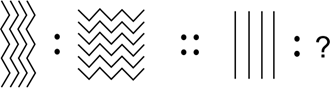a)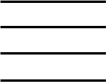b)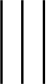c)d)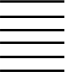Q.2 Which among the following will replace the question mark?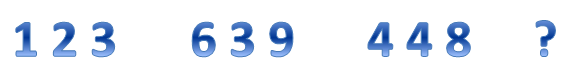a)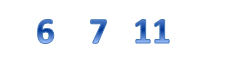b)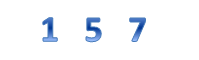c)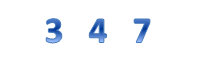d)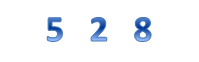Q.3 Which object is same as the given figure?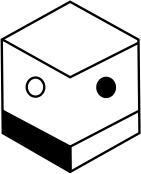a)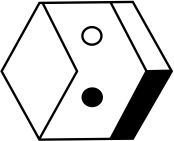b)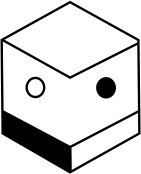c)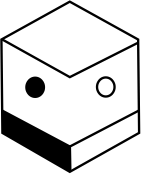d)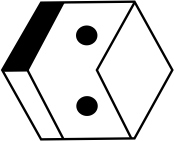Q.4 Neha has 10 chocolates. She gives 2 chocolates to Pooja and 2 to Sohan. Roopali gives Neha 5 chocolates. How many chocolates does Neha have? a) 10 b) 9 c) 11 d) 8
 Q.5 Which among the following will you select to complete the pattern?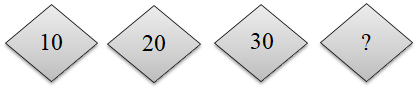a)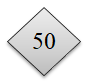b)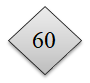c)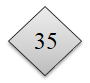d)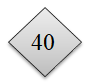Q.6 Tabriz was having boiled eggs and an omelet for breakfast? What according to you is the shape of the omelet and that of the egg? a) Egg is circle and omelet is circle. b) Egg is oval and omelet is square. c) Egg is oval and omelet is circle. d) Egg is circle and omelet is oval.
 Q.7 Which figure/pattern/shape comes next?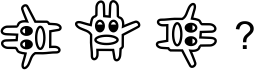a)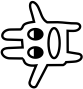b)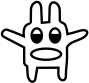c)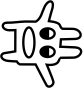d)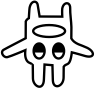Q.8 Which object is same as the given figure?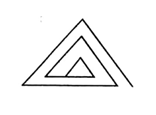a)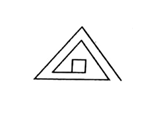b)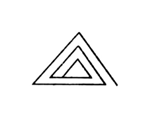c)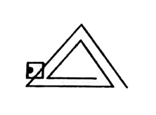d)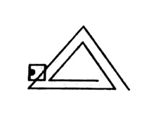Q.9 There is a certain relationship between the pair of figures given on either side of (::) identify the relationship of given pair and find the matching term.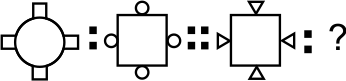a)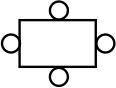b)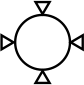c)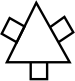d)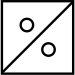Q.10 Which group does Figure X belong to?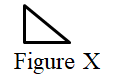a)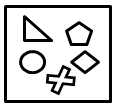Group A b)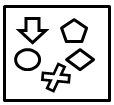Group B c)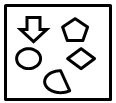Group C d)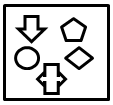Group DSample PDF of CREST Olympiads - CREST Reasoning Olympiad (CRO) PDF Sample Papers for Class 1: